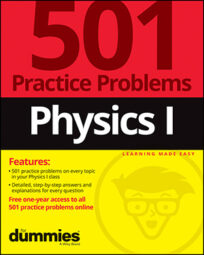##### Physics I: 501 Practice Problems For Dummies (+ Free Online Practice)When solving physics problems, you’ll often encounter unit prefixes that you need to know. You also need to be familiar with common unit symbols and their corresponding SI units. The following tables list some of the typical prefixes and symbols that you may see.

Commonly Used Unit Prefixes in Physics Problems
Unit Prefix Name Symbol Factor
tera- T 1012
giga- G 109
mega- M 106
kilo- k 103
centi- c 10–2
milli- m 10–3
micro- ì 10–6
nano- n 10–9
pico- p 10–12
Handy Unit Conversions for Physics Problems
Derived Units Symbol SI Units
Newton N kilogram meter per second squared
Joule J kilogram meter squared per second squared
Watt W kilogram meter squared per second cubed
Hertz Hz inverse second
Pascal P kilogram per meter per second squared
Degree Celsius °C kelvin